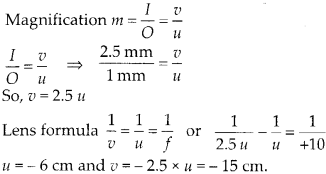Enlightened

# Question 31: NCERT Solutions for 12th Class Physics: Chapter 9-Ray Optics and Optical Instruments

• 0

Question 31: NCERT Solutions for 12th Class Physics: Chapter 9-Ray Optics and Optical Instruments

What should be the distance between the object in previous question and the magnifying glass if the virtual image of each square in the figure is to have an area of 6.25 mm2. Would you be able to see the squares distinctly with your eyes very close to the magnifier?

Share

1. Solution:
Now we want the area of square shaped virtual image as 6.25 mm2. So, each side of image is I == 2.5 mm (Linear magnification) For the given magnifying lens of focal length 10 cm we can calculate the required position of object.Thus the required virtual image is closer than normal near point. Thus the eye cannot observe the image distinctly.

Check the complete chapter with solutions.

NCERT Solutions for 12th Class Physics: Chapter 9-Ray Optics and Optical Instruments

• 0